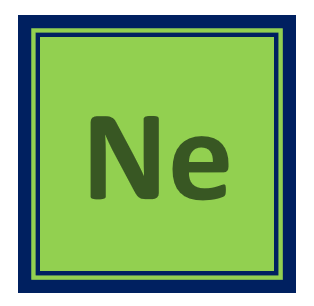## Physical properties of sulfur dioxide gas:

Sulfur dioxide is a colorless gas at room temperature.

It has a pungent odor that is similar to the smell of burnt matches.

Sulfur dioxide is soluble in water, and it reacts with water to form sulfurous acid (H2SO3).

The boiling point of sulfur dioxide is -10°C (-50°F), and the melting point is -75°C (-103°F).

## Chemical properties of sulfur dioxide gas:

Sulfur dioxide is a highly reactive gas that readily undergoes a variety of chemical reactions.

It is a reducing agent, which means it is capable of transferring electrons to other molecules.

Sulfur dioxide can react with many metals, such as iron, to form metal sulfites.

It can also react with many organic compounds to form sulfite esters, which are commonly used in the production of fragrances and flavors.

Sulfur dioxide can act as an acid and react with bases to form sulfites.

## Uses of sulfur dioxide gas:

Sulfur dioxide is used as a preservative in the food industry to prevent the growth of bacteria and fungi.

It is used in the production of sulfuric acid, which is an important industrial chemical used in a variety of processes.

Sulfur dioxide is used as a reducing agent in many chemical reactions.

It is also used as a bleach in the production of paper and textiles.

Sulfur dioxide is used in the wine industry to control the growth of spoilage microorganisms and to prevent the oxidation of wine.

## Reactions of sulfur dioxide gas with other compounds:

Sulfur dioxide can react with water to form sulfurous acid (H2SO3), which is a weak acid that can further react with bases to form sulfites.

It can react with metals, such as iron, to form metal sulfites.

Sulfur dioxide can also react with organic compounds to form sulfite esters, which are commonly used in the production of fragrances and flavors.

When sulfur dioxide is burned in the presence of oxygen, it forms sulfur trioxide (SO3), which is an important precursor to the production of sulfuric acid.

## Equations for the reactions of sulfur dioxide gas with other compounds

### Reaction with oxygen gas:

SO2(g) + 1/2O2(g) → SO3(g)

### Reaction with water to form sulfurous acid:

SO2(g) + H2O(l) → H2SO3(aq)

### Reaction with calcium oxide to form calcium sulfite:

SO2(g) + CaO(s) + H2O(l) → CaSO3(s) + H2O(l)

### Reaction with hydrogen sulfide to produce elemental Sulfur:

SO2(g) + 2H2S(g) → 3S(s) + 2H2O(g)

### Reaction with chlorine gas to form sulfur dichloride:

SO2(g) + Cl2(g) → SO2Cl2(l)

### Reaction with ammonia gas to form ammonium sulfite:

SO2(g) + 2NH3(g) + H2O(l) → (NH4)2SO3(s)

## How to produce Sulfur dioxide gas:

Sulfur dioxide gas can be produced through the burning of sulfur-containing fuels, such as coal or oil, or by roasting sulfide ores. It can also be produced through the reaction of sulfur trioxide with water.

Here are some examples of reactions that can be used to produce sulfur dioxide gas:

### Burning sulfur in air:

S(s) + O2(g) → SO2(g)

### Roasting metal sulfide ores:

2FeS2(s) + 11O2(g) → 2Fe2O3(s) + 4SO2(g)

### Reaction of metal sulfites with an acid:

CaSO3(s) + 2HCl(aq) → CaCl2(aq) + H2O(l) + SO2(g)

### Reaction of metal sulfites with oxygen:

2Na2SO3(s) + O2(g) → 2Na2SO4(s)

### Reaction of metal sulfides with oxygen:

4FeS(s) + 7O2(g) → 2Fe2O3(s) + 4SO2(g)

### Thermal decomposition of metal sulfites:

2CuSO3(s) → 2CuO(s) + 3O2(g) + 2SO2(g)

## Sulfur dioxide lewis structure

The Lewis structure of sulfur dioxide (SO2) can be drawn as follows:By <a href=”//commons.wikimedia.org/wiki/User:EatePurple” title=”User:EatePurple”>EatePurple</a> – <span class=”int-own-work” lang=”en”>Own work</span>, CC0, Link

In this structure, sulfur is the central atom and it is bonded to two oxygen atoms with a double bond. Each oxygen atom is also bonded to sulfur with a single bond. The sulfur atom has six valence electrons, while each oxygen atom has six valence electrons. This structure shows that sulfur dioxide has two lone pairs on the sulfur atom and one lone pair on each oxygen atom.

## Sulfur dioxide molar mass

The molar mass of sulfur dioxide (SO2) can be calculated by adding up the atomic masses of one sulfur atom and two oxygen atoms.

### Sulfur dioxide molar mass calculation

The atomic mass of sulfur (S) is 32.06 g/mol, and the atomic mass of oxygen (O) is 16.00 g/mol.

Therefore, the molar mass of SO2 can be calculated as:

Molar mass of SO2 = (1 x atomic mass of S) + (2 x atomic mass of O)

= (1 x 32.06 g/mol) + (2 x 16.00 g/mol)

= 64.06 g/mol

Hence, the molar mass of sulfur dioxide is approximately 64.06 g/mol.